Budget-Aware Regularization (BAR) allows to simultaneously train and prune a neural network architecture, while respecting a neuron budget. The method is targeted at “turning off” irrelevant feature maps in CNNs. To find which feature maps are relevant, “Dropout Sparsity Learning” is used. To respect the budget, a novel barrier function is introduced.

Contributions at a glance:

• Objective function that optimizes/constrains the total number of neurons
• Novel barrier optimization method
• Mixed-Connectivity Block that leverages atypical connectivity

# Dropout Sparsity Learning

We learn which feature maps to keep by using a special kind of dropout. The first difference with traditional dropout is that each feature map is associated with a different Bernoulli parametrization (e.g. probability of being active). Let’s call this “feature-wise dropout”.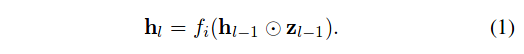$$\mathbf{z}$$ is a vector of “dropout variables”. The goal is to learn the parametrization of each dropout variable, with a preference for parameterizations that turn off the feature map. However, ordinary feature-wise dropout, because of its discrete nature, does not allow to learn the parameters by backpropagation. We will thus use a continuous relaxation of Bernoulli, named the Hard Concrete distribution. Using the Reparametrization Trick, we can draw samples from the distribution like so:

\begin{aligned} z &= g(\Phi_{l-1},\epsilon) \\ z &\in [0,1] \\ \epsilon &\sim \mathcal{U}(0,1) \end{aligned}

We rewrite Eq. (1) like this: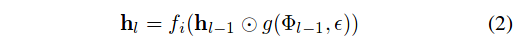The following is a plot of the function $$g(\Phi,\epsilon)$$ associated with the Hard Concrete. For our purposes, $$\Phi := \alpha$$.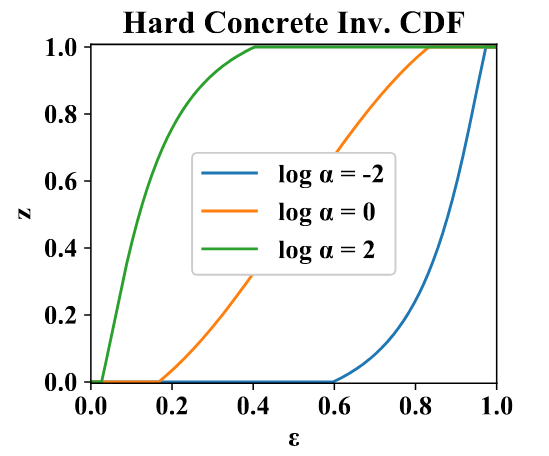Here is an intuition about this plot. Take the blue curve; it is associated to a irrelevant feature map. Why? Imagine you draw samples $$\epsilon \sim \mathcal{U}(0,1)$$ and you give them to this function. It will mostly output zero; in fact $$P(z=0) \approx 0.6$$.

The interpretation of $$\alpha$$ is that it controls $$P(z=0)$$, $$P(z=1)$$, and the probability density in between (for $$z \in~]0,1[~$$). The weird thing with the Hard Concrete, is that while there is virtually no chance of picking a specific value, for example $$z=0.5$$ (why 0.5 when you could have 0.50001 ?), there is a substantial probability of picking exactly $$z=0$$ (or $$z=1$$). Why should we give a $$\int u \subset k$$, you ask? Well, we’d like $$z$$ to be zero as much as possible, since this corresponds to a pruned feature map. But if $$z$$ is close to zero but non-zero, the signal can still go through; the next layer could scale it up to a useful range. Thus, picking exactly zero is important1.

To sparsify the network, we will minimize $$P(z>0)$$ for all $$z$$, for which we have a closed-form expression $$L_S(\Phi)$$.

# Budget-Aware Regularization

To promote the removal of neurons, a sparsity term is used in the loss. To enforce the budget, a novel barrier function $$f(V,a,b)$$ is used: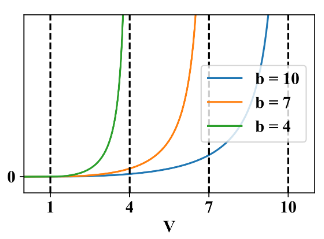where $$V$$ is the volume of the activation maps2 and $$b$$ is the budget. For this figure, $$a=1$$; this hyperparameter corresponds to the value of $$V$$ where the budget is comfortably respected. The barrier function is multiplied with the sparsity loss: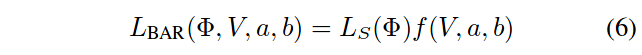During training, $$b$$ is transitioned from a value larger than the initial $$V$$, to $$B$$, the “final budget”.

The complete loss function is the sum of a data term and the sparsity loss term (above). The data term can be a cross-entropy, but a Knowledge Distillation objective is suggested for easier pruning.

# Mixed-Connectivity Block

By pruning feature maps in residual blocks, atypical connectivity emerges: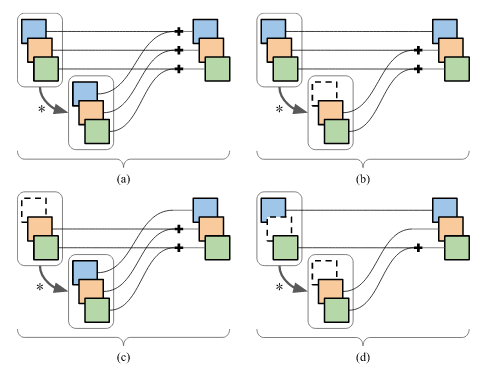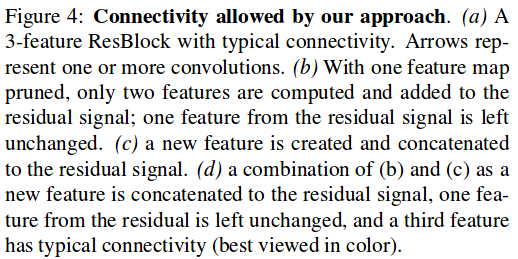These connectivity patterns generally do not provide computational savings, unless they are leveraged by a special Mixed-Connectivity block implementation (given in the paper).

# Results

At the highest pruning factors, this method outperforms the state of the art. It also requires less hyperparameter tuning.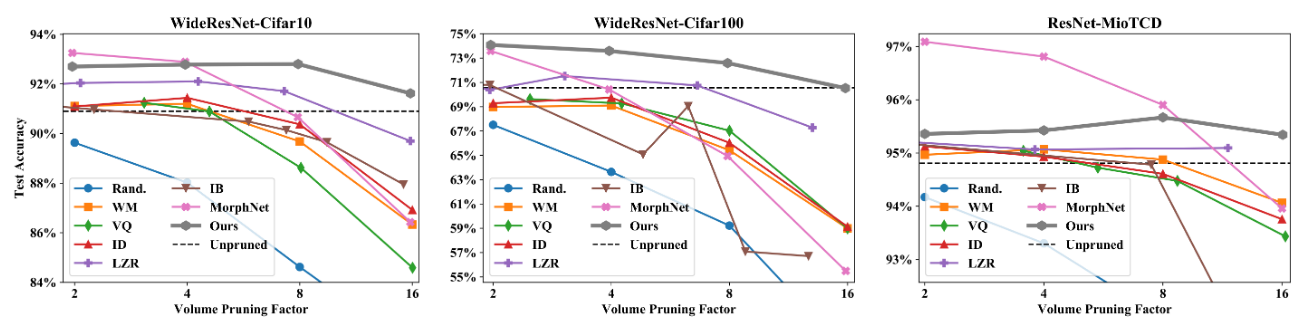1. Picking exactly one is nice also (it would mean that a feature map is REALLY important). But it’s not as crucial as $$P(z=0) \gg 0$$.

2. $$V = \sum_l \sum_i \mathbf{1}(\mathbb{E}[z_{l,i} \vert \Phi] > 0) \times A_l$$ where $$l$$ is the layer, $$i$$ is the feature map and $$A_l$$ is the area of the feature maps.